# RD Sharma Solutions for Class 10 Maths Chapter 9 Arithmetic Progression Exercise 9.4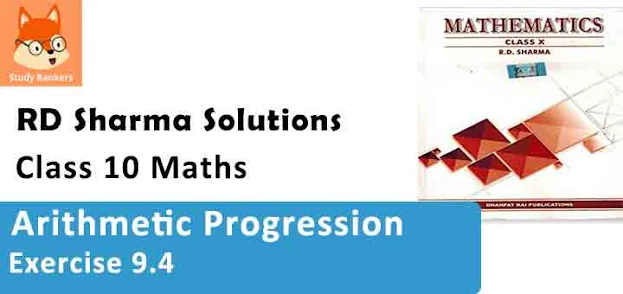1. Find:

(i) 10th term of the A.P. 1, 4, 7, 10, .......
(ii) 18th term of A.P. √2, 3√2, 5√2, .......
(iii) nth term of the A.P. 13, 8, 3, -2, ......
(iv) 10th term of the A.P. -40, -15, 10, 35, .....
(v) 8th term of the A.P. 11, 104, 91, 78, .......
(vi) 11th term of the A.P. 10.0, 10.5, 11.0, 11.5, .....
(vii) 9th term of the A.P. 3/4, 5/4, 7/4, 9/4 , .......

Solution

(i) Given A.P. is
1, 4, 7, 10, ......
First term (a) = 1
Common difference (d) = second term - first term
= 4 - 1 = 3
nth term in an A.P = a (n -1)d
10th term in an A.P = 1 + (10 - 1)3
= 1 + 9×3
= 1 + 27 = 28

(ii) Given, A.P is √2, 3√2, 5√2, .......
First term (a) = √2
common difference(d) = second term - first term
3√2 - √2 = 2√2
n th term in an A.P = a + (n -1)d
18 th term of A.P = √2 + (18 - 1)2√2
= √2 + 17 × 2√2
= √2(1 + 34)
= 35√2

(iii) Given, A.P is 13, 8, 3, -2, .......
First term (a) = 13
Common difference (d) = Second term - first term
8 - 13 = - 5
nth term of an A.P = a + (n -1)d
= 13 + (n -1) - 5
= 13 - 5n + 5
= 18 - 5n

(iv) Given A.P is
-40, -15, 10, 35, .......
First term (a) = -40
Common difference (d) = Second term - first term
= -15 - (-40)
= 40 - 15 = 25
nth term of an A.P = a + (n -1)d
10th term of A.P = -40 + (10 - 1)25
= -40 + 9×25
= -40 + 225 = 185

(v) Given sequence is
117, 104, 91, 78, .........
First learn can = 117
Common difference (d) = Second term - first term
= 104 - 117 = - 13
nth term = a + (n -1)d
8th term = a + (8 - 1)d
= 117 + 7(-13)
= 117 - 91 = 26

(vi) Given A.P is 10.0, 10.5, 11.0, 11.5, .....
First term (a) = 10.0
Common difference (d) = Second term - First term
= 10.5 - 10.0 = 0.5
nth term = a + (n - 1)d
11th term = 10.0 + (11 - 1) 0.5
= 10.0 + 10 ×0.5
= 10.0 + 5= 15.0

(vii) Given A.P. is 3/4, 5/4, 7/4, 9/4 , ........
First term (a) = 3/4
Common difference (d) = Second term - First Term
= 5/4 - 3/4 = 2/4
nth term = a + (n - 1)d
9th term = a + (9 -1)d
= 3/4 + 8× 2/4
= 3/4 + 16/4
= 19/4

2. (i) Which term of the AP 3, 8, 13, ..... is 248?
(ii) Which term of the AP 84, 80, 76, ...... is 0 ?
(iii) Which term of the AP 4, 9, 14, ...... is 254 ?
(iv) Which term of the AP 21, 42, 63, 84, ...... is 420?
(v) Which term of the AP 121, 117, 113, ...... is its first negative term ?

Solution

(i) Given A.P is 3, 8, 13, .....
First term (a) = 3
common difference (d) = Second term - first term
= 8 - 3 = 5
n th term (an) = a + (n - 1)d
Given nth term an = 248
248 = 3 + (n - 1)5
⇒ 248 = -2 + 5n
⇒ 5n = 250
⇒ n = 250/5 = 50
50th term is 248

(ii) Given A.P is 84, 80, 76, .......
First term (a) = 84
Common difference (d) = a2 - a
= 80 - 84 = -4
nth term (an) = a + (n - 1)d
Given nth term is 0
0 = 84 + (n - 1)× -4
⇒ 0 = 84 -4(n - 1)
⇒ 4(n-1) = 84
⇒ n - 1= 84/4 = 21
⇒ n = 21 + 1= 22
⇒ 22 nd term is 0.

(iii) Given A.P. 4, 9, 14, .......
First term (a) = 4
Common difference (d) = a2 - a
= 9 - 4 = 5
nth term (an) = a + (n -1)d
Given nth term is 254
4 + (n - 1)5 = 254
⇒ (n - 1)5 = 250
⇒ n - 1= 250/5 = 50
= 50 + 1 = 51
∴ 51 th term is 254.

(iv) Given A.P 21, 42, 63, 84, ......
a = 21, d = a2 - a
= 42 - 21 = 21
nth term (an) = a + (n - 1)d
Given nth term = 420
21 + (n - 1)21 = 420
⇒ (n - 1)21 = 399
⇒ n - 1 = 399/21 = 19
⇒ n = 19 + 1 = 20
∴ 20th term is 420.

(v) Given A.P is 121, 117, 113,........
First term (a) = 121
Common difference (d) = 117 - 121 = -4
nth term (a) = a + (n - 1)d
Given nth term is negative i.e., an < 0
121 + (n - 1)-4 < 0
⇒ 121+ 4 - 4n < 0
⇒ 125 < 4n
⇒ n > 125/4
⇒ n > 31.25
The integer which comes after 31.25 is 32,
∴ 32 nd term is first negative term.

3. (i) Is 68 a term of the AP 7, 10, 13, ...... ?
(ii) Is 302 a term of the AP 3, 8, 13,...... ?
(iii) Is -150 a term of the AP 11, 8, 5, 2, ...... ?

Solution

In the given problem, we are given an A.P and the value of one of its term
We need to find whether it is a term of the AP or not so here we will use the formula
an = a + (n - 1)d

(i) Here, AP is 7, 10, 13, ......
an = 68, a = 7, and d = 10 - 7 = 3
Using the above mentioned formula, we get
68 = 7 + (n - 1)3
⇒ 68 - 7 = 3n - 3
⇒ 31 + 3 = 3n
⇒ 64 = 3n
⇒ n = 64/3
Since, the value of n is a fraction.
Thus, 68 is not the team of the given A.P.

(ii) Here, AP is 3, 8, 13,....
an = 302, a = 3
d = 8 - 3= 5 using the above mentioned formula, we get
302 = 3 + (n -1)5
⇒ 302 - 3 = 5n - 5
⇒ 299 = 5n - 5
⇒ 5n = 304
⇒ n = 304/5
Since, the value of 'n' is a fraction. Thus, 302 is not the term of the given A.P.

(iii) Here, A.P. is 11, 8, 5, 2, ......
an = -150, a = 1 and d = 8 - 11= -3
Thus, using the above mentioned formula, we get
-150 = 11 + (n - 1)(-3)
⇒ -150 - 11 = -3n + 3
⇒ -161 - 3 = -3n
⇒ n = -164/-3
Since, the value of n is a fraction. Thus, -150 is not the term of the given A.P.

4. How many term are there in the AP ?
(i) 7, 10, 13,..... 43
(ii)-1, -5/6, -2/3, -1/2,....., 10/3
(iii) 7, 13, 19,...... 05
(iv) 18, 15(1/2), 13, ...... , -47

Solution

(i) 7, 10, 13,..... 43
From given A.P
a = 7, d = 10 - 7 = 3, an = a + (n - 1)d.
Let, an = 43 (last term)
7 + (n - 1)3 = 43
⇒ (n-1) = 39/3=13
⇒ n = 13
∴ 13 terms are there in given A.P.

(ii) -1, -5/6, -2/3, -1/2,........, 10/3
From given A.P
a = -1, d = -5/6 + 1 = 1/6
an = a + (n - 1)d
Let, an = 10/3 (last term)
-1 + (n - 1)1/6 = 10/3
⇒ (n - 1) × 1/6 = 13/3
⇒ n - 1 = (13 × 6)/3 = 26
⇒ n = 27
∴ 27 terms are there in given A.P.

(iii) 7, 13, 19, ....., 05
From the given A.P
a = 7, d = 13 - 7 = 6,
an = a + (n - 1)d
Let an = 205 (last term)
7 + (n - 1)6 = 205
⇒ (n - 1)6 = 205- 7 = 198
⇒ n -1 = 198/6 = 33
⇒ n = 33+ 1 = 34
∴ 34 terms are there in given A.P.

(iv) 18, 15(1/2), 13, ...... , -47
From the given A.P
a = 18, d = 31/2 - 18 = 15.5 - 18 = -2.5
an = a + (n - 1)d
Let an = -47  (last term)
18 + (n - 1) × -2.5 = - 47
⇒ (n -1)× -2.5 = -47 - 18
⇒ n - 1= -65/-2.5
⇒ n - 1 = (65 × 10)/25 = 26
⇒ n = 26 + 1 = 27
∴ 27 terms are there in given A.P.

5. The first term of an AP is 5, the common difference is 3 and the last term is 80, find the number of terms.

Solution

Given,
First term(a) = 5
Common difference (d) = 3
Last term (an ) = 80
To calculate no of terms in given A.P
an = a + (n - 1)d
Let an = 80,
80 = 5 + (n - 1)3
⇒ (n - 1)3 = 75
⇒ n - 1 = 75/3 = 25
⇒ n = 25 + 1 = 26
∴ There are 26 terms.

6. The 6th and 17 terms of an A.P are 19 and 41 respectively, find the 40th term.

Solution

Given, a6 = 19, a17 = 41
⇒ a6 = a + (6 - 1)d
19 = a + 5d  ...(1)
an = a + (17 - 1)d
⇒ 41 = a + 16d ...2)
Subtract (1) from (2)
a + 16d = 41 ...(1)
a + 5d = 19 ...(2)
11d = 22
⇒ d = 22/11 = 2
Substitute d = 2 in (1)
19 = a + 5(2)
a = 9
∴ 40th term a40 = a + (40 - 1)d
= 9 + 39×2
= 9 + 78 = 87
∴ a40 = 87

7. If 9th term of an A.P is zero, prove that its 29th term is double the 19th term.

Solution

Given,
9th term of an A.P a9 = 0,
an = a + (n - 1)d
a + (a - 1)d = 0
⇒ a + 8d = 0
⇒ a = -8d
We have to prove,
24th term is double the 19th term a29 = 2. a19
a + (29 - 1)d = 2[a + (19 - 1)d]
a + 28d = 2[a + 18d]
Put a = -8d
-8d + 28d = 2[-8d + 18d]
⇒ 20d = 2×10d
⇒ 20d = 20d
Hence, proved.

8. If 10 times the 10th term of an A.P is equal to 15 times the 15th term, show that 25th term of the A.P. is zero.

Solution

Given,
10 times of 10th term is equal to 15 times of 15th term.
10a10 = 15.a15
10[a + (10 - 1)d] = 15[a + (15 - 1)d] [∴ an = a + (n - 1)d]
⇒ 10(a + 9d) = 15(a + 14.d)
⇒ a + 9d = 15/10(a + 14d)
⇒ a - (3/2)a = 42d/2 - 9d
⇒ -1a/2 = (42d - 18d)/2
⇒ -a/2 = 24d/2 =12d
⇒ a = -24d
We have to prove 25 th term of A.P is 0
a25 = 0
⇒ a + (25 - 1)d = 0
⇒ a + 24d = 0
Put a = -24d
-24d + 24d = 0
⇒ 0 = 0
Hence proved.

9. The 10th and 18th terms of an A.P. are 41 and 73 respectively. Find 26th term.

Solution

Given,
an = 41, an = 73,
an = a +(n - 1)d
⇒ a10 = a + (10 - 1)d
41 = a + 9d  ...(1)
⇒ an = a + (18 - 1)d
73 = a + 17d  ...(2)
Subtract (1) from (2)
a + 17d = 73  ...(1)
⇒ a + 9d = 41  ...(2)
⇒ 0 + 8d = 32
⇒ d = 32/8 = 4
Substitute d = 4 in (1)
a + 9×4 = 41
⇒ a = 41 - 36
⇒ a = 5
26th term an = a + (26 - 1)d
= 5 + 25×4
= 5 + 100
= 105
∴ 26th term a26 = 105.

10. In a certain A.P the 24th term is twice the 10th term. Prove that the 72 nd term is twice the 34th term.

Solution

Given,
24th term is twice the 10th term
a24 = 2 a10
Let, first term of a square = a
Common difference = d
nth term an = a + (n - 1)d
a + (24 - 1)d = (a + (10 - 1)d
⇒ a + 23d = 2(a + 9d)
⇒ (23 - 18)d = a
⇒ a = 5d
We have to prove,
72nd term is twice the 34th term
a12 = 2a34
⇒ a + (12 - 1)d = 2[a + (34 - 1)d]
⇒ a + 71d = 2a + 66d
Substitute a = 5d
5d + 71d = 2(5d) + 66d
⇒ 76d = 10 d + 66d
⇒ 76d = 76d
Hence proved.

11. If (m + 1)th term of an A.P. is twice the (n + 1)th term, prove that (3m + 1)th term is twice the (m + n +1)th term.

Solution

Given,
(m + 1) th term is twice the (m + 1)th term.
First term = a
Common difference = d
n th term an = a + (n - 1)d
am+1 = 2an + 1
⇒ a + (m + 1 - 1)d = 2(a + (n + 1 - 1)d
⇒ a + md = 2(a + nd)
⇒ a = (m - 2n)d
We have to prove
(3m + 1)th term is twice the (m + n + 1)th term
a3m + 1 = 2.am+n+1
⇒ a + (3m + 1 - 1)d = (a + (m + n + 1 -1)d
⇒ a + 3m.d - 2a + 2(m + n)d
Substitute a = (m - 2n)d
(m - 2n)d + 3m d = 2(m - 2n)d + 2(m + n)d
⇒ 4m - 2n = 4m - 4n + 2n
⇒ 4m - 2n = 4m - 2n
Hence, proved.

12. If the n term of the A.P 9, 7, 5, ...... is same as the term of the A.P. 15, 12, 9, ..... find n.

Solution

Given,
First sequence is 9,7,5, .....
a = 9, d = 7 - 9 = -2, an = a + (n + 1)d
an = 9 + (n - 1)×-2
Second sequence is 15, 12, 9, .....
a = 15, d = 12 - 15 = -3, an = a + (n - 1)d
an = 15 + (n - 1) × -3
Given an an are equal
9 -2(n - 1) = 15 - 3(n - 1)
⇒ 3(n - 1) - 2(n - 1) - 15 - 9
⇒ n - 1 = 6
⇒ n = 7
∴ 7th term of two sequence are equal.

13. Find the 12th term from the end of the following arithmetic progressions:
(i) 3, 5, 7, 9, .....201
(ii) 3, 8,13, ......, 253
(iii) 1, 4,7, 10, ...... 88

Solution

(i) 3, 5, 7, 9, ....., 201
First term (a) = 3
Common difference (d) = 5 - 3 = 2
12th term from the end is can be considered as (l) last term = first term and common difference = d1 = -d
nth term from the end = last term + (n - 1)×-d
12 th term from end = 201 + (12 - 1)× -2
= 201 - 22
= 179

(ii) 3, 8, 13, .....,253
First term a = 3
Common difference d = 8 - 3 = 5
Last term (l) = 253
n th term of sequence on = a + (n - 1)d
To find nth term from the end, we put last term (l) as 'a' and common difference as -d
nth term from the end = last term + (n - 1) -d
12th term from the end = 253 + (12 - 1) ×-5
= 253 - 55 = 198
∴ 12 th term from the end = 198

(iii) 1, 4, 7, 10, ......., 88
First term a = 1
Common difference d = 4 - 1 = 3
Last term (l) = 88
n th term an = a + (n + 1)d
n th term from the end = last term + (n - 1)-d
12 th term from the end = 88 + (12 - 1)× -3
= 88 - 33
= 55
∴ 12th term from the end = 55

14. The 4th term of an A.P is three times the first and the 7 term exceeds twice the third term by 1. Find the first term and the common difference.

Solution

Given,
4 th term of an AP is three times the times the first term
a4 = 3.a
n th term of a sequence an = a + (n - 1)d
a + (4 - 1)d = 3a
⇒ a + 3d = 3a
⇒ 3d = 2a
⇒ a = 3d/2  ...(1)
Seventh term exceeds twice the third term by 1.
a7 + 1 = 2a3
⇒ a + (7 - 1)d + 1 = 2[a+(3 -1)d]
⇒ a + 6d + 1 = 2a + 4d
⇒ 2a - a = 6d - 4d + 1
⇒ a = 2d + 1  ...(2)
By equating (1), (2)
3d/2 = 2d + 1
⇒ 3d/2 - 2d = 1
⇒ -d/2 = 1
⇒ d = -2
Put d = -2 in a = 3d/2
= (3/2)(-2)
= -3
∴ First term a = -3, common difference d = -2.

15. Find the second term and nth term of an A.P. whose 6th term is 12 and the 8th term is 22.

Solution

Given,
a6 = 12, a8 = 22
nth term of an A.P an = a + (n - 1)d
a6 = a + (6 - 1)d = a + 5d = 12 ...(1)
a8 = a+ (8 -1)d = a + 7d = 22  ...(2)
Subtracting (1) from (2)
2d = 10
⇒ d = 5
putting value of d in equation (1)
a + 5d = 12
⇒ a + 5×5 = 12
⇒ a = 12 - 25 = -13
Second term a2 = a + (2 - 1)d
=a + d
= -13 + 5 = -8
n th term an = a + (n - 1)d
= -13 + (n - 1)×5
= -13 + 5n - 5
= -18 + 5n
nth term an = a + (n - 1)d
= -13 + (n - 1)-5
an = -18 + 5n
∴ a2 = -8, an = -18 + 5n

16. How many number of two digits are divisible by 3 ?

Solution

We observe that 12 is the firs two - digit number divisible by 3 and 99 is the last two digit number divisible by 3. Thus, the sequence is
12, 15, 18,..., 99
This sequence is in A.P with
First term (a) = 12
Common difference(d) = 15 - 12 = 3
nth term an = 99
nth term of an A.P
(an) = a + (n -1)d
99 = 12 +(n -1)3
⇒ 99 - 12 = (n - 1)3
⇒ 87/3 = n = 1
⇒ n = 30
∴ 30 term are there in the sequence.

17. An A.P. consists of 60 terms. If the first and the last terms be 7 and 125 respectively, find 32 nd term.

Solution

Given,
No. of terms = n = 60
First term (a) = 7
Last term (a) = 7
Last term (a10) = 125
an = a + (n - 1)d
⇒ a60 = a + (60 - 1)d
⇒ 125 = 7 + 59d
⇒ 118 = 59d
⇒ d = 118/59 = 2
52 nd term a32 = a + (32 - 1)d
= 7 + 31×2
= 7 + 62 = 69

18. The sum of 4 and 8th terms of an A.P. is 24 and the sum of the 6th and 10 th terms is 34. Find the first term and the common difference of the A.P.

Solution

Given,
a4 + a8 = 24
a6 + a10 = 34
⇒ a + (4 - 1)d + a + (18 - 1)d = 24
2a + 10d = 24
a + 5d = 12 ....(1)
⇒ a6 + a10 = 34
a + (6 - 1)d + a + (10 - 1)d = 34
⇒ 2a + 14d = 34
a + 7d = 17 ....(2)
Subtract (1) from (2)
a + 7d = 17
⇒ a + 5d = 12
⇒ 2d = 5
⇒ d = 5/2
Put d = 5/2 in a + 5d = 12
a = 12 - 5d
⇒ a = 12 - 5× 5/2
⇒ a = 12 - 25/2 = -1/2
∴ a = -1/2, d = b/2

19. The first term of an A.P. is 5 and its 100th term is -292. Find the 50th term of this A.P.

Solution

Given,
First term of an A.P. = 5 and 100th term = -292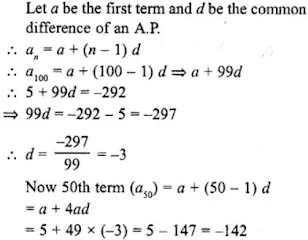20. Find a30 – a20 for the A.P.
(i) -9, -14, -19, -24, …
(ii) a, a + d, a + 2d, a + 3d, …

Solution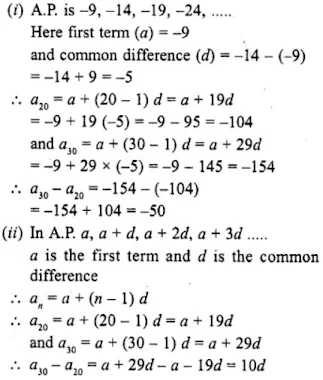21. Write the expression an – ak for the A.P. a, a + d, a + 2d, ……
Hence, find the common difference of the A.P. for which
(i) 11th term is 5 and 13th term is 79.
(ii) a10 – a5 = 200
(iii) 20th term is 10 more than the 18th term.

Solution

In the A.P. a, a + d, a + 2d, ….
an = a+(n-1)d and ak = a+(k-l)d
an - ak = [a + (n-1)d] - [a + (k-1)d]
= a + nd - d - (a + kd - d)
= a + nd - d - a - kd + d
= nd - kd - (n - k)d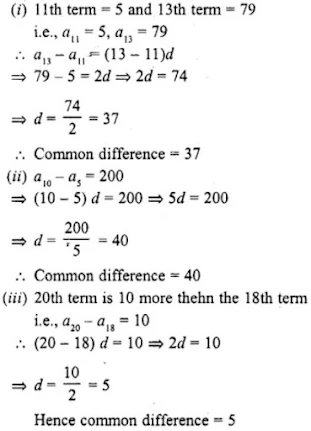22. Find n if the given value of x is the nth term of the given A.P.

(i) 25, 50, 75, 100,....; x = 1000
(ii) -1, -3, -5, -7, ....; x = -151
(iii) 5½, 11, 16½, 22, ....; x = 550
(iv) 1, 21/11, 31/11, 41/11, ....; x = 171/11

Solution

(i) The A.P. is 25, 50, 75, 100, ...; x = 1000
Here, a = 25 and d = 50 - 25 = 25 and an = 100
an = a+(n-1)d
1000= 25 + (13-1)×25
= 25 + 25n - 25 = 25n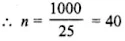(ii) The A.P. is -1, -3, -5, -7, ...; x = -151
Here, a = -1, d = -3 -(-1) = -3 + 1 = -2 and an = -15
an = a + (n-1)d
⇒ -151 = -1 + (n-1)(-2)
⇒ -151 = -1 - 2n + 2
⇒ -151 = 1 - 2n
⇒ -2n = -151 - 1 = -152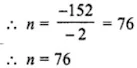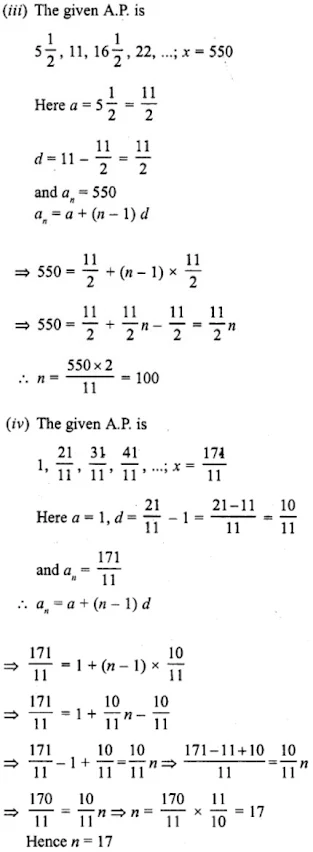23. The eighth term of an A.P. is half of its second term and the eleventh term exceeds one third of its fourth term by 1. Find the 15th term.

Solution

Let a and d be the first term and common difference of an A.P., respectively.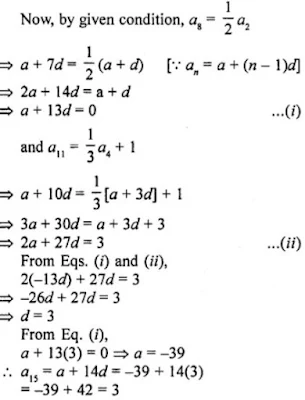\

24. Find the arithmetic progression whose third term is 16 and seventh term exceeds its fifth term by 12.

Solution

Let a, a + d, a + 2d, a + 3d, …. be the A.P.
an = a + (n – 1) d
But a3 = 16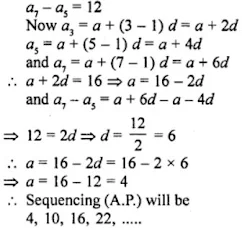25. The 7th term of an A.P. is 32 and its 13th term is 62. Find the A.P.

Solution

Let a, a + d, a + 2d, a + 3d, be the A.P.
Here a is the first term and d is the common difference
an = a + (n – 1) d
Now, a7 = a + (7 – 1) d = a + 6d = 32 ….(i)
and a13 = a + (13 – 1) d = a + 12d = 62 ….(ii)
Subtracting (i) from (ii)
6d = 30
⇒ d = 5
a + 6×5 = 32
⇒ a + 30 = 32
⇒ a = 32 – 30 = 2
A.P. will be 2, 7, 12, 17, ……

26. Which term of the A.P. 3, 10, 17, … will be 84 more than its 13th term ?

Solution

The given A.P. is 3, 10, 17,... whose first term (a) = 3 and,
common difference (d) = 10 - 3 = 7
an = a + (n-1)d
Let kth term is greater than 13th term by 84.
kth term(ak) = a + (k-1)d
and a13 = a + (13-1) = a + 12d
But ak - a13 = 84
a + (k-1)d - (a + 12d) = 84
⇒ a + kd - d - a - 12d = 84
⇒ kd - 13d = 84
⇒ (k-13)d = 84
⇒ 7(k-13) = 84
⇒ 7k - 91 = 84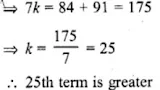27. Two arithmetic progressions have the same common difference. The difference between their 100th terms is 100, what is the difference between their 1000th terms ?

Solution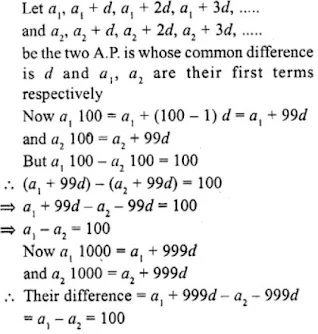28. For what value of n, the nth terms of the arithmetic progressions 63, 65, 67, ...... and 3, 10, 17, ..... are equal ?

Solution

Given two A.P is are:
63, 65, 67,.... and 3, 10, .....
In the A.P. 63, 65, 67, …
a = 63 and d = 65 – 63 = 2
an = a1 + (n–1) d = 63 + (n–1)×2 = 63 + 2n – 2 = 61 + 2n
and in the A.P. 3, 10, 17, …
a = 3 and d = 10 – 3 = 7
an = a + (n–1) d = 3 + (n–1)×7 = 3 + 7n – 7 = 7n – 4
But both nth terms are equal
61 + 2n = 7n – 4
⇒ 61 + 4 = 7n – 2n
⇒ 65 = 5n
⇒ n = 13

29. How many multiples of 4 lie between 10 and 250 ?

Solution

All the terms between 10 and 250 are multiple of 4
First multiple (a) = 12
and last multiple (l) = 248
and d = 4
Let n be the number of multiples, then
an = a + (n–1) d
⇒ 248 = 12 + (n–1)×4 = 12 + 4n – 4
⇒ 248 = 8 + 4n
⇒ 4n = 248 – 8 = 240
n = 60
Number of terms are = 60

30. How many three digit numbers are divisible by 7 ?

Solution

First three digit number is 100 and last three digit number is 999
In the sequence of the required three digit numbers which are divisible by 7, will be between
a = 105 and last number l = 994 and d = 7
Let n be the number of terms, then
an = a + (n – 1) d
994 = 105 + (n–1)×7
⇒ 994 = 105 + 7n – 7
⇒ 7n = 994 – 105 + 7
⇒ 7n = 896
⇒ n = 128
Number of terms =128

31. Which term of the arithmetic progression 8, 14, 20, 26, … will be 72 more than its 41st term ?

Solution

Let nth term is 72 more than its 41 st term
an = a41 + 72
For the given sequence
a = 8, d = 14 - 8 = 6
⇒ a + (n - 1)d = 8 + (n -1)6 + 72
⇒ 8 + (n - 1)6 = 8 + 40×6 + 72
⇒ (n - 1)6 = 312
⇒ n - 1 = 312/6 = 52
⇒ n = 52+1 = 53
∴ 53rd term is 72 more than 41st term

32. Find the term of the arithmetic progression 9, 12, 15, 18, … which is 39 more than its 36th term.

Solution

In the given A.R 9, 12, 15, 18, …
First term (a) = 9
and common difference (d) = 12 – 9 = 3
and an = a + (n – 1) d
Now a36 = a + (36 – 1) d = 9 + 35×3 = 9 + 105 = 114
Let the an be the required term
an = a + (n – 1) d
= 9 + (n – 1)×3 = 9 + 3n – 3 = 6 + 3n
But their difference is 39
an – a36 = 39
⇒ 6 + 3n – 114 = 39
⇒ 114 – 6 + 39 = 3n
⇒ 3n = 147
⇒ n = 49
Required term is 49th.

33. Find the 8th term from the end of the A.P. 7, 10, 13, …, 184.

Solution

The given A.P. is 7, 10, 13,…, 184
Here first term (a) = 7
and common difference (d) = 10 – 7 = 3
and last tern (l) = 184
Let nth term from the last is an = l – (n–1) d
a8= 184 – (8–1)×3 = 184 – 7×3 = 184 – 21 = 163

34. Find the 10th term from the end of the A.P. 8, 10, 12, …, 126.

Solution

The given A.P. is 8, 10, 12, …, 126
Here first term (a) = 8
Common difference (d) = 10 – 8 = 2
and last tern (l) = 126
Now nth term from the last is an = l – (n–1) d
a10 = 126 – (10–1)×2
= 126 – 9×2
= 126 – 18
= 108

35. The sum of 4th and 8th terms of an A.P. is 24 and the sum of 6th and 10th terms is 44. Find the A.P.

Solution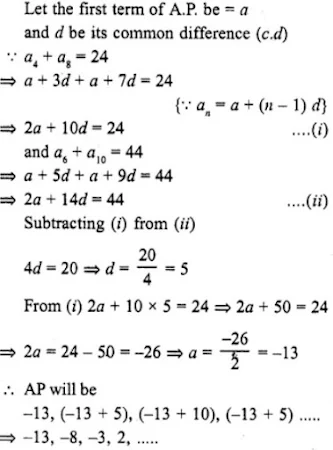36. Which term of the A.P. 3, 15, 27, 39, ..... will be 120 more than its 21st term?
Solution
Given, A.P. is 3, 15, 27, 39, .....
Here first term (a) = 3 and c.d. (d) = 15 - 3 = 12
Let nth term be the required term
Now,
21st term = a + (n – 1) d = 3 + 20 x 12 = 3 + 240 = 243
According to the given condition,
nth term – 21 st term = 120
⇒ a + (n – 1) d – 243 = 120
⇒ 3 + (n – 1)×12 = 120 + 243 = 363
⇒ (n – 1) 12 = 363 – 3 = 360
⇒ n – 1 = 30
⇒ n = 30 + 1 = 31
31st term is 120 more than 21st term.

37. The 17th term of an A.P is 5 more than twice its 8th term. If the 11th term of the A.P. is 43, find the nth term.

Solution

Given,
17th term of an A.P is 5 more than twice its 8th term.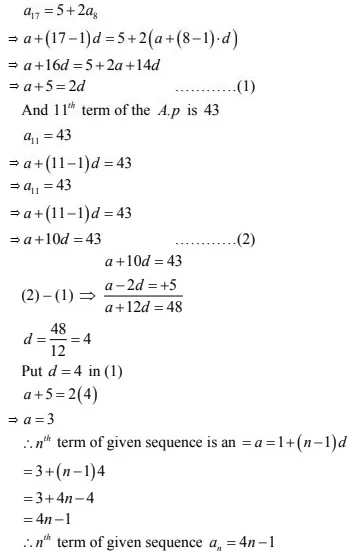38. Find the number of ail three digit natural numbers which are divisible by 9.
Solution
First 3 digit number which is divisible by 9 = 108 and last 3 digit number = 999
d= 9
a + (n – 1) d = 999
⇒ 108 + (n – 1)×9 = 999
⇒ (n – 1) d = 999 – 108
⇒ (n – 1)×9 = 891
⇒ n – 1 = 99
⇒ n = 99 + 1 = 100
Number of terms = 100

39. The 19th term of an A.P. is equal to three times its sixth term. If its 9th term is 19, find the A.P.
Solution
Let a be the first term, d be the common difference, then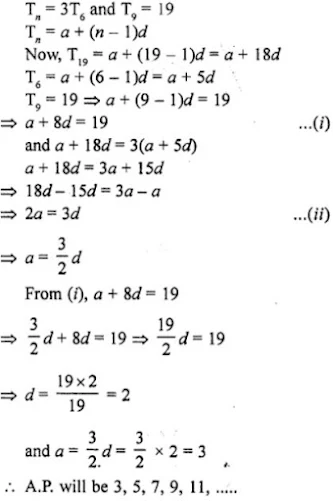40. The 9th term of an A.P. is equal to 6 times its second term. If its 5th term is 22, find the A.P.

Solution

Let a be the first term and d be the common difference and
Tn = a + (n–1) d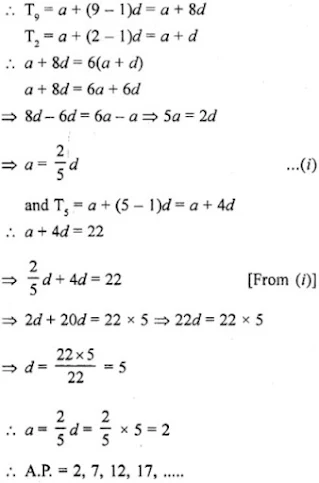41. The 24th term of an A.P. is twice its 10th term. Show that its 72nd term is 4 times its 15th term.

Solution

Let a be the first term, d be the common difference, then
Tn = a + (n–1) d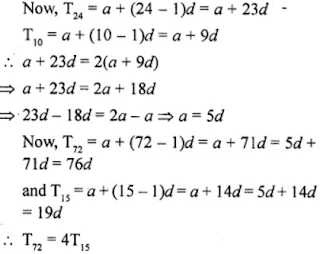Hence, 72nd term = 4 times of 15th term.

42. Find the number of natural numbers between 101 and 999 which are divisible by both 2 and 5.

Solution

Numbers divisible by both 2 and 5 are 110, 120, 130, ………. , 990
Here a = 110, x = 120 – 110 = 10
an = 990
A,
a + (n – 1) d = 990
⇒ 110 + (n – 1) (10) = 990
⇒ (n – 1) (10) = 990 – 110 = 880
⇒ n – 1 = 88
⇒ n = 88 + 1 = 89

43. If the seventh term of an AP is 1/9 and its ninth term is 1/7, find its 63rd term.

Solution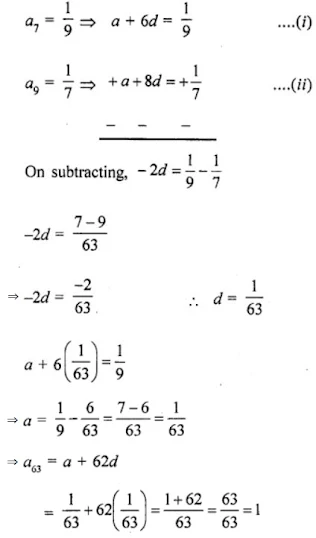44. The sum of 5th and 9th terms of an AP is 30. If its 25th term is three times its 8th term, find the AP.
Solution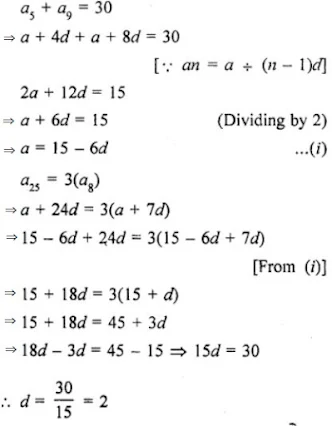From (i),
a = 15 - 6(2) = 15 - 12 = 3
A.P. is a, a+d, a+2d, a+3d,....
⇒ 3, 5, 7, 9,....

45. Find where 0 (zero) is a term of the AP 40, 37, 34, 31, ……

Solution

AP 40, 37, 34, 31, …..
Here, a = 40, d = -3
Let Tn = 0
Tn = a + (n – 1) d
⇒ 0 = 40 + (n – 1) (-3)
⇒ 0 = 40 – 3n + 3
⇒ 3n = 43
⇒ n = 433 which is in fraction.
There is no term which is 0.

46. Find the middle term of the A.P. 213, 205, 197, …, 37.

Solution

A.P. is 213, 205, 197,....37
Here,
a = 213, d = 205-213 = -8 and l = 37
Let number of terms = n, then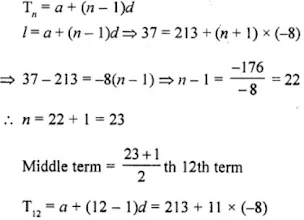= 213 - 88 = 125
∴ Middle term = 125.

47. If the 5th term of an A.P. is 31 and 25th term is 140 more than the 5th term, find the A.P.

Solution

We know that,
Tn = a + (n – 1 )d
T5 = a + 4d
⇒ a + 4d = 31 …(i)
and T25 = a + 24d
⇒ a + 24d = 140 + T5
⇒ a + 24d = 140 + 31 = 171 …(ii)
Subtracting (i) from (ii),
20d= 140
and a + 4d = 31
⇒ a + 4×7 = 31
⇒ a + 28 = 31
⇒ a = 31 – 28 = 3
a = 3 and d = 7
AP will be 3, 10, 17, 24, 31, ….

48. Find the sum of two middle terms of the A.P.
-4/3, -1, -2/3, -1/3,...., 4 1/3

Solution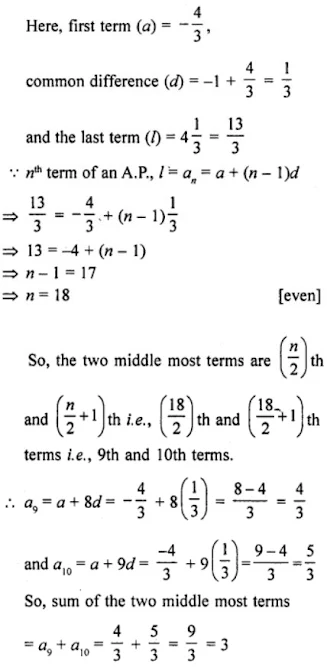49. If (m + 1)th term of an A.P. is twice the (n + 1)th term, prove that (3m + 1)th term is twice the (m+n+1)th term.

Solution

Let a, a+d, a+2d, a+3d, .... is in A.P.
∴ (m+1)th term = a + (m+1-1)d
= a + md
and,
(n+1)th term = a + (n+1-1)d
= a + nd
∵ (m+1)th term = 2(n+1)th term
∴ a + md = 2(a + nd)
⇒ a + md = 2a + 2nd
⇒ 2a - a = md - 2 nd
⇒ a = d(m - 2n) = (m - 2n) d ...(i)
Now,
(3m + 1)th term = a+(3m + 1 - 1) d
= (m - 2n) d + 3md
= (m - 2n + 3m)d
= (4m - 2n)d
= 2 (2m - n) d ...(ii)
and (m+n+1)th term = a+(m+n+1-1)d
= a+(m+n)d
= (m-2n)d + (m+n)d
= (m-2n+m+n)d
= (2m - n)d ...(iii)
From (ii) and (iii),
(3m + 1)th term = 2 (m + n + 1)th term.
Hence, proved.

50. If an A.P. consists of n terms with first term a and nth term l show that the sum of the mth term from the beginning and the mth term from the end is (a + l).

Solution

In an A.P.,
Number of terms = n
First term = a
and nth term = l
mth term (am) = a + (m – 1) d
and mth term from the end = l – (m – 1)d
Their sum = a + (m – 1) d + l – (m – 1) d = a + l
Hence proved.

51. How many numbers lie between 10 and 300, which when divided by 4 leave a remainder 3?

Solution

Here, the first number is 11, which divided by 4 leave remainder 3 between 10 and 300.
Last term before 300 is 299, which divided by 4 leave remainder 3.
11, 15, 19, 23, …, 299
Here, first term (a) = 11,
common difference (d) = 15 – 11 = 4
nth term, an = a + (n – 1 ) d = l [last term]
⇒ 299 = 11 + (n – 1) 4
⇒ 299 – 11 = (n – 1) 4
⇒ 4(n – 1) = 288
⇒ (n – 1) = 72
n = 73

52. Find the 12th term from the end of the A.P. -2, -4, -6, …, -100.

Solution

Given, A.P., -2, -4, -6, …, -100
Here, first term (a) = -2,
common difference (d) = -4 - (-2)
and the last term (l) = -100.
We know that, the nth term an of an A.P. from the end is an = l - (n - 1 )d,
where l is the last term and d is the common difference. 12th term from the end,
an = -100 - (12 - 1) (-2)
= -100 + (11) (2)
= -100 + 22
= -78
Hence, the 12th term from the end is -78.

53. For the A.P.: -3, -7, -11,…, can we find a30 – a20 without actually finding a30 and a20 ? Give reasons for your answer.

Solution

True.
nth term of an A.P., an = a + (n – 1)d
a30 = a + (30 – 1 )d = a + 29d
and a20 = a + (20 – 1 )d = a + 19d …(i)
Now, a30 – a20 = (a + 29d) – (a + 19d) = 10d
and from given A.P.
common difference, d = -7 – (-3) = -7 + 3 = -4
a30 – a20 = 10(-4) = -40 [from Eq- (i)]

54. Two A.P.s have the same common difference. The first term of one A.P. is 2 and that of the other is 7. The difference between their 10th terms is the same as the difference between their 21st terms, which is the same as the difference between any two corresponding terms. Why?

Solution

Let the same common difference of two A.P.’s is d.
Given that, the first term of first A.P. and second A.P. are 2 and 7 respectively,
then the A.P.’s are 2, 2 + d, 2 + 2d, 2 + 3d, … and 7, 7 + d, 7 + 2d, 7 + 3d, …
Now, 10th terms of first and second A.P.’s are 2 + 9d and 7 + 9d, respectively.
So, their difference is 7 + 9d – (2 + 9d) = 5
Also, 21st terms of first and second A.P.’s are 2 + 20d and 7 + 20d, respectively.
So, their difference is 7 + 20d – (2 + 9d) = 5
Also, if the an and bn are the nth terms of first and second A.P.
Then bn - an = [7 + (n - 1) d] – [2 + (n - 1) d = 5
Hence, the difference between any two corresponding terms of such A.P.’s is the same as the difference between their first terms.